# Weight Mass Volume and Density What is Weight

• Slides: 19Weight, Mass, Volume and Density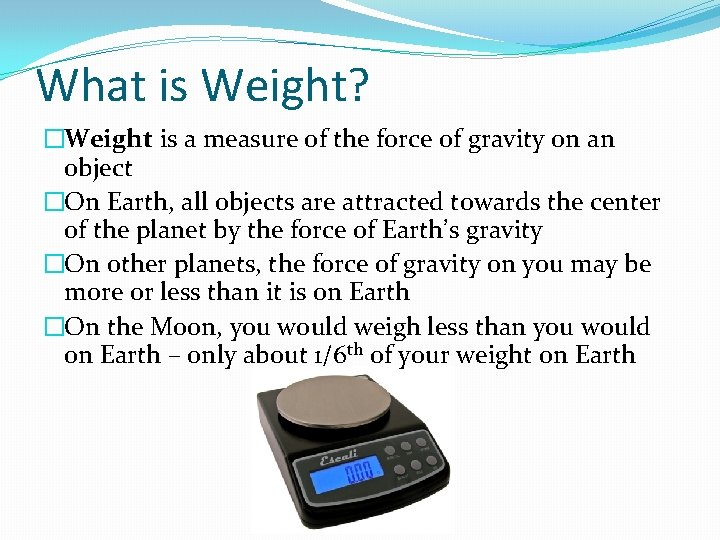What is Weight? �Weight is a measure of the force of gravity on an object �On Earth, all objects are attracted towards the center of the planet by the force of Earth’s gravity �On other planets, the force of gravity on you may be more or less than it is on Earth �On the Moon, you would weigh less than you would on Earth – only about 1/6 th of your weight on EarthWhat is Mass? �The force of gravity depends partly on the mass of an object �The mass of an object is the measurement of the amount of matter in the objectWhy do you weigh less on the moon? �If you travel to the moon, your mass does not change �The mass of the moon is much less than the mass of Earth �Therefore the moon will exert much less gravitational force on you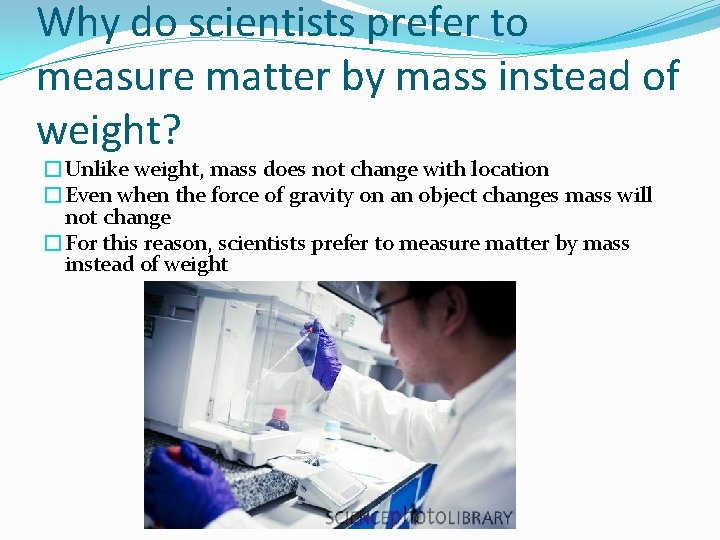Why do scientists prefer to measure matter by mass instead of weight? �Unlike weight, mass does not change with location �Even when the force of gravity on an object changes mass will not change �For this reason, scientists prefer to measure matter by mass instead of weight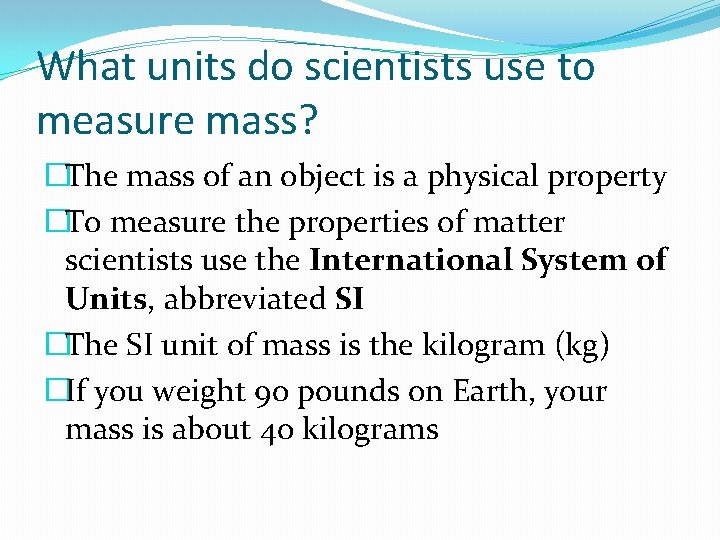What units do scientists use to measure mass? �The mass of an object is a physical property �To measure the properties of matter scientists use the International System of Units, abbreviated SI �The SI unit of mass is the kilogram (kg) �If you weight 90 pounds on Earth, your mass is about 40 kilograms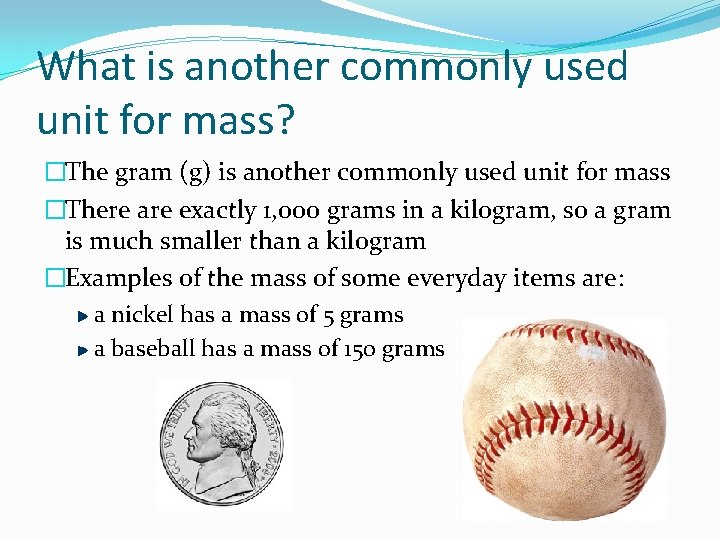What is another commonly used unit for mass? �The gram (g) is another commonly used unit for mass �There are exactly 1, 000 grams in a kilogram, so a gram is much smaller than a kilogram �Examples of the mass of some everyday items are: a nickel has a mass of 5 grams a baseball has a mass of 150 grams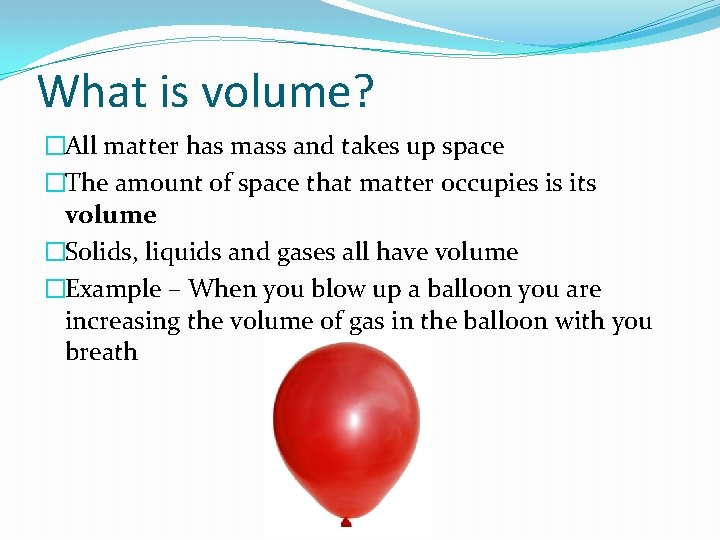What is volume? �All matter has mass and takes up space �The amount of space that matter occupies is its volume �Solids, liquids and gases all have volume �Example – When you blow up a balloon you are increasing the volume of gas in the balloon with you breath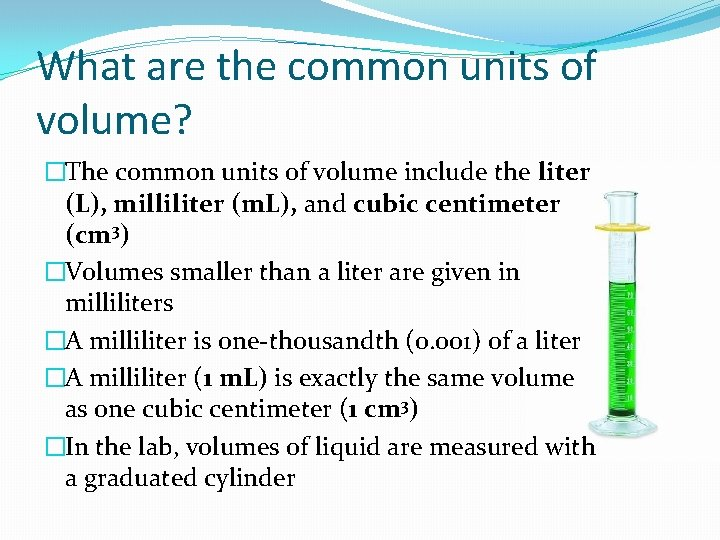What are the common units of volume? �The common units of volume include the liter (L), milliliter (m. L), and cubic centimeter (cm 3) �Volumes smaller than a liter are given in milliliters �A milliliter is one-thousandth (0. 001) of a liter �A milliliter (1 m. L) is exactly the same volume as one cubic centimeter (1 cm 3) �In the lab, volumes of liquid are measured with a graduated cylinder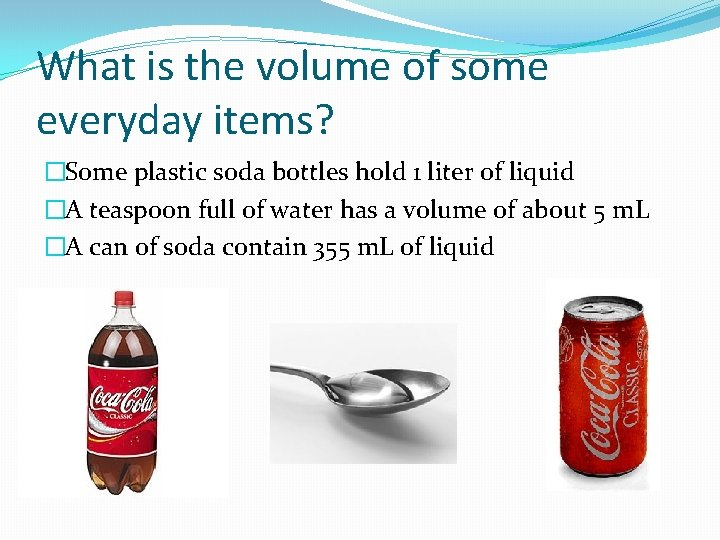What is the volume of some everyday items? �Some plastic soda bottles hold 1 liter of liquid �A teaspoon full of water has a volume of about 5 m. L �A can of soda contain 355 m. L of liquidHow do you calculate the volume of a solid rectangular object? �The volumes of solid objects are usually expressed in cm 3 �If you want to know the volume of a rectangular object, such as a brick, you need to measure the object’s length, width, and height, then, you multiply these valuesCalculating Volume of a solid rectangular object �Volume = Length x Width x Height Width Length �Measurements always have units, so when you multiply the 3 measurements, you must multiply the units as well as the numbers Units = cm x cm = cm 3How can you measure the volume of an irregularly shaped object? �The most commonly used way to measure the volume of an irregularly shaped object, such as a rock, is to use the water displacement method �First you fill up a graduated cylinder with a level of water that will cover the object �Then you drop the irregularly shaped object into the graduated cylinder of water and observe how much higher the water level becomesHow can you measure the volume of an irregularly shaped object? �The volume of the object would be the difference between the volume of the object in the water from the volume of just the water �Subtracting these two volumes will give you the volume of an irregularly shaped object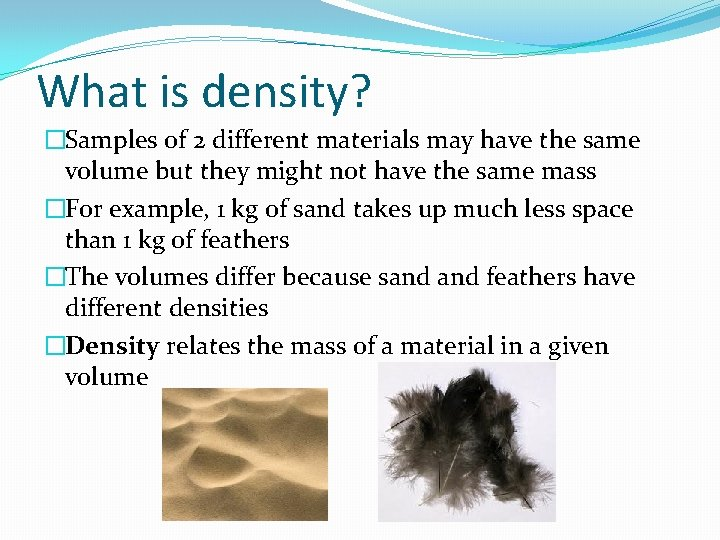What is density? �Samples of 2 different materials may have the same volume but they might not have the same mass �For example, 1 kg of sand takes up much less space than 1 kg of feathers �The volumes differ because sand feathers have different densities �Density relates the mass of a material in a given volume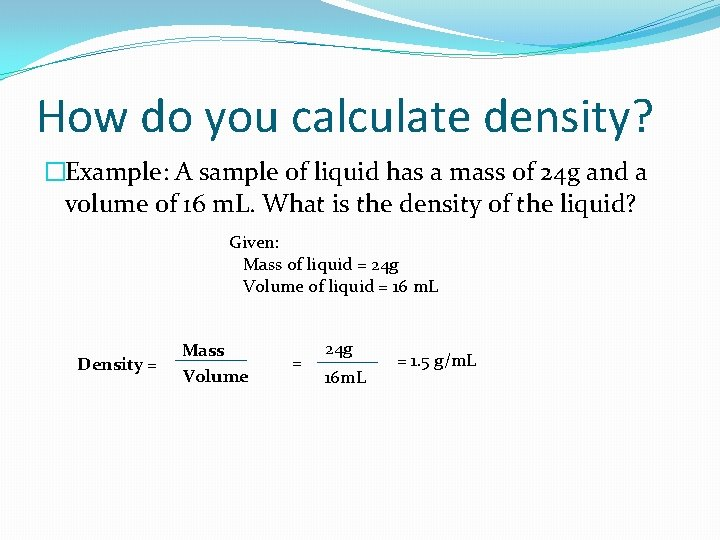How do you calculate density? �Example: A sample of liquid has a mass of 24 g and a volume of 16 m. L. What is the density of the liquid? Given: Mass of liquid = 24 g Volume of liquid = 16 m. L Density = Mass Volume = 24 g 16 m. L = 1. 5 g/m. LHow is density expressed? �Density is expressed as the number of grams in one cubic centimeter �For example, the density of water at room temperature is stated as “one gram per cubic centimeter (1 g/cm 3) �You can determine the density of a sample of matter by dividing its mass by its volume Density = Mass VolumeHow do we use density? �Whether or not an object sinks or floats depends on its density �For example, if we drop a solid block of wood and a solid block of iron into a tub of water, we would see that the wood would float while the iron would sinkHow do we use density? �Since the density of water is 1 g/cm 3 , any object that is greater than the density of water, such as iron, will sink �Any object that is less than the density of water, such as wood, will float �Density is a physical property of a substance that can be used to identify an unknown substance